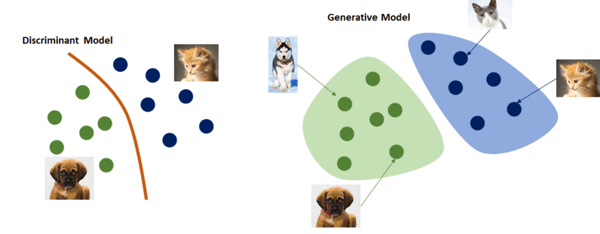a shot of dev knowledge

Related tags

# Generative vs. discriminative model

Let’s look at a scenario to understand both of theseGenerative model and Discriminative model models.

Imagine you are a superhero, but instead of saving humans, you are supposed to save all the apples in dangerthey are being attacked by insects. Let’s say you are an apple-man, sent on a mission to save all the apples on earth from insects.

Now, imagine that there are just two fruits on earth, apples and bananas, but you do not want to save the bananas because they are your enemy. There is one problem, you have never seen an apple or a banana in real-life. However, since your childhood, your father has shown you pictures of apples and bananas, which have has given you two superpowers:

1. From all the pictures that you have seen since childhood, you could draw an image of an apple yourself. Then, you could compare your drawn image with reality to determine whether or not the fruit is an apple. This “superpower” is known as the generative model.

2. Based on the physical differences that you can recognize (i.e., color, shape, etc.), you can determine if something is a banana or an apple. This “superpower” is known as the discriminative model.### Discriminative model

1. A Discriminative Model uses a decision boundary between classes (as shown above).

2. Discriminative models learn the conditional probability distribution p(y|x).

3. Discriminative models are used in supervised learning algorithms.

4. These models directly use training data to predict parameters of p(y|x).

5. Examples include Logistic regression, Scalar Vector Machine, Traditional neural networks, Nearest neighbor, and Conditional Random Fields (CRF)s.

### Generative model

1. A Generative Model uses an actual distribution to model each class.

2. Generative models learn the joint probability distribution p(x,y). They use Bayes theorem to predict the conditional probability.

3. Generative models are used in supervised learning algorithms.

4. These models directly use the training data to predict parameters of p(y|x).

5. Examples include Naive Bayes, Bayesian networks, Markov random fields, and Hidden Markov Models (HMM).

References:

1. Image taken from medium.

Related tags

RELATED COURSES
Related Courses
Related Courses

#### Keep Exploring

Learn in-demand tech skills in half the time

Copyright ©2021 Educative, Inc. All rights reserved.